# How to Add Words/Texts from Different Cells Together in Excel?

When we want to combine the data of many cells into one cell, we can use the concept of formulas in Excel. We can add the data of two or more cells into one cell in one of two ways: by using the keyword CONCAT or by using the "&" symbol in Excel. In general, we use this method to create data sets or person details. In this tutorial, let's understand the concepts behind adding words or texts from different cells together in Excel.

## Add Words/Texts from Different Cells Together Using "&"

Here, we will first use the formula to get our first result, then use the auto-fill handle to complete the task. Let us see the process of adding texts from different cells together using the "&" symbol.

Step 1

Consider the following Excel sheet with data similar to the image below.

To get the first result, click on an empty cell, type =A2&B2&C2&D2 into it, and press Enter.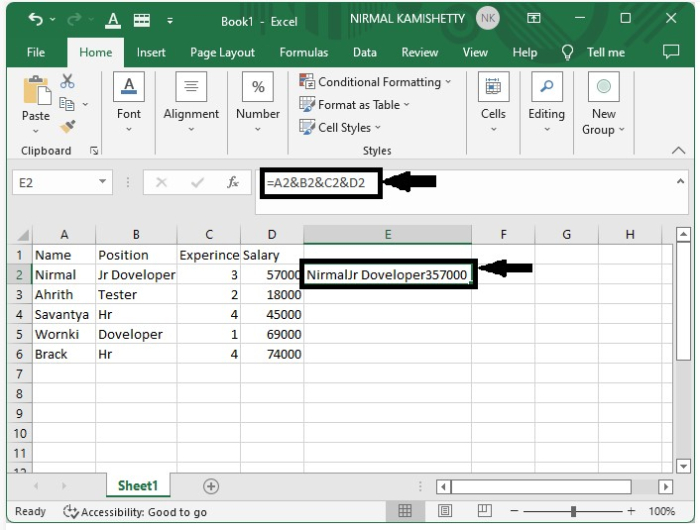As we can see, the data is very messy. We can solve this problem by adding "," in between the formulas so that it becomes =A2&","&B2&","&C2&","&D2" and clicking on enter. Then the data will be neatly separated by commas.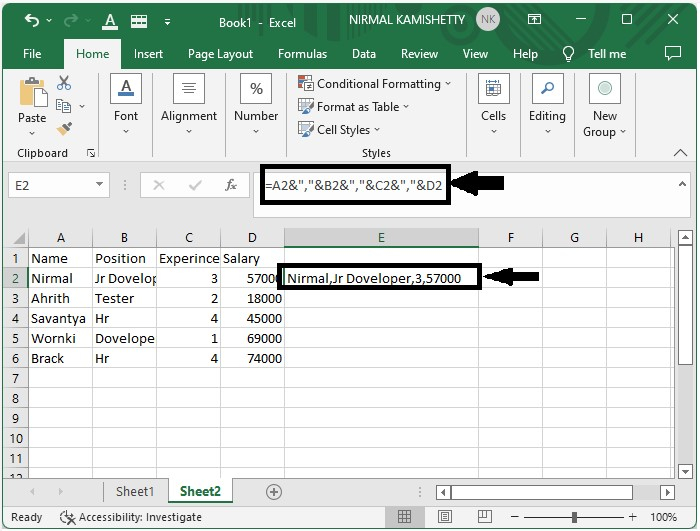Step 2

Now we can get the other results by dragging down from the result that is already present, and the final result will be shown as the below image.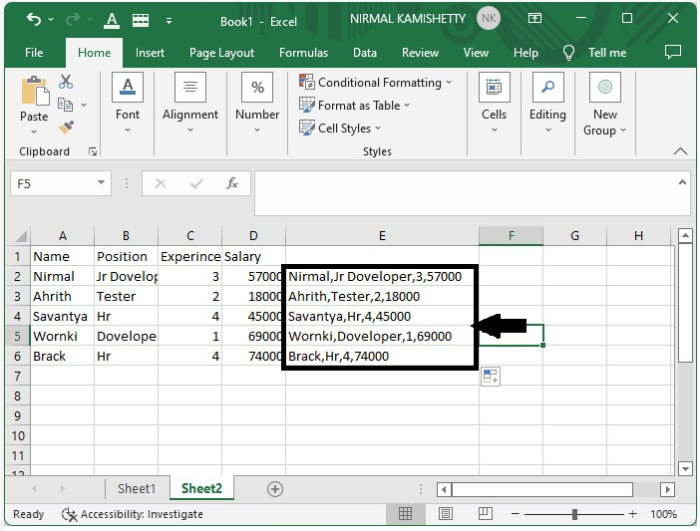## Add Words/Texts from Different Cells Together Using CONCAT

Here, we will first use the CONCAT formula to get the first result, then use the auto-fill handle to complete the task. Let's go over how to use the CONCAT function to combine the words.

Step 1

Consider the same example as in the previous one.

To get the first result, select the empty cell and enter the formula =CONCAT(A2,",",B2,",",C2,",",D2 in the formula box and press Enter.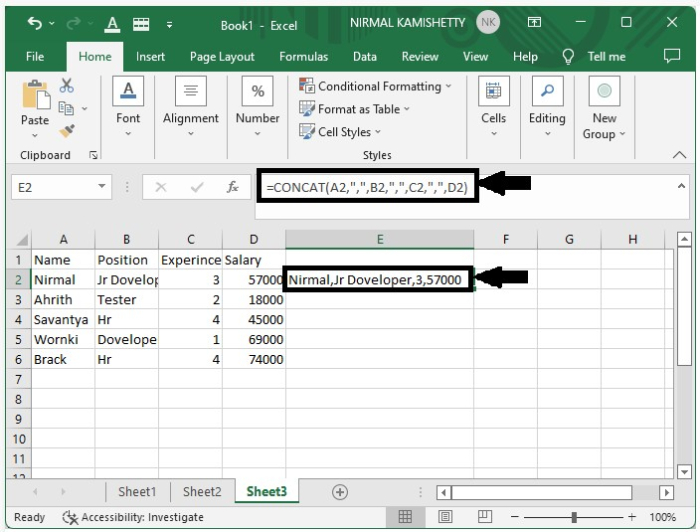Step 2

Now, drag down from the first result to fill in all the remaining results.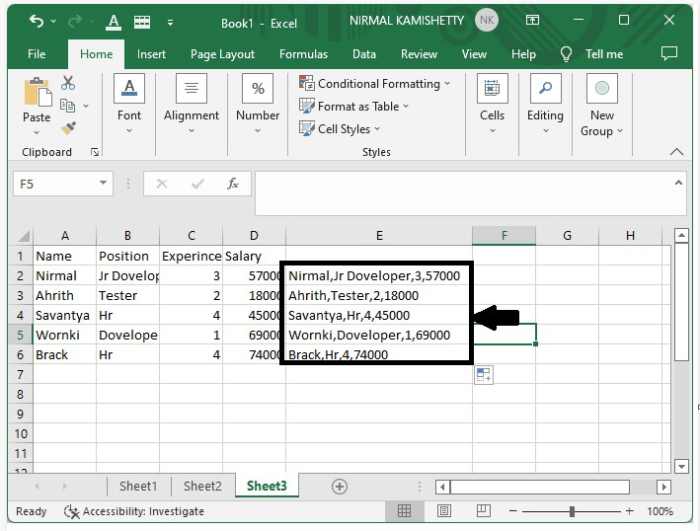## Conclusion

In this tutorial, we used a simple example to demonstrate how we can add the contents of multiple cells into one cell in Excel to highlight a particular set of data.

Updated on: 09-Jan-2023

116 Views